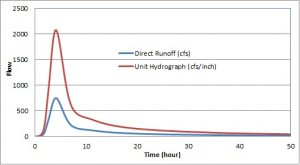# What is Unit Hydrograph in Water Resource Engineering

The unit hydrograph of a watershed is defined as a direct runoff hydrograph resulting from 1 unit of excess rainfall generated uniformly over the drainage area at a constant rate for an effective duration.The unit hydrograph is a simple linear model that can be used to derive the hydrograph resulting from any amount of excess rainfall.

The following basic assumptions are inherent in this model;

1. Rainfall excess of equal duration are assumed to produce hydrographs with equivalent time bases regardless of the intensity of the rain

2. Direct runoff ordinates for a storm of given duration are assumed directly proportional to rainfall excess volumes.

3. The time distribution of direct runoff is assumed independent of antecedent precipitation

4. Rainfall distribution is assumed to be the same for all storms of equal duration, both spatially and temporally

Open